# Quiz 13: Fiscal Policy, Deficits, and Debt

The government spending would be magnified by the multiplier effects. We first calculate multiplier by using MPC.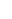Then we calculate government spending.By tax cut, consumption increases by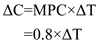From the steps above, to bring the same change in demand, the change in consumption equals to change in government spending.For the combination of spending raise and tax cut, let us suppose government spending raise by G, and tax was cut by T. The total effect should increase initial spending by \$5 billion, and the multiplier effect would push the total demand by \$25 billion.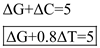Any Government expenditure and Tax that meet the above equation can be a combination. For example, if tax cut is \$5 billion, then government spending increase should be \$1 billion. It showed as below: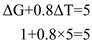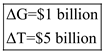Real Gross Domestic Production ( GDP ) refers to the total value of all final goods and services produced given a period of time or in a year. Aggregate Demand refers to the total amount of goods and services demanded in the economy at a given general price level and given a period of time. Table of increases in Aggregate Demand: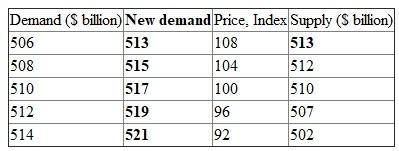The new equilibrium price level iS108, where the new demand equals the supply. Before the change the equilibrium price level waS100. The price level increases by 8%. It showed as below: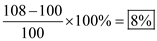The inflation is caused by an increase in demand; thus, it is a Demand-pull Inflation. If the potential GDP IS \$510 billion and the equilibrium GDP is \$513 billion, there is an inflationary GDP gap of \$3 billion. The government should implement contractionary fiscal policy to eliminate the inflation. It can reduce government spending.